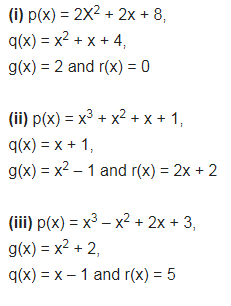# Give examples of polynomials p(x), g(x), q(x) and r(x), which satisfy the division algorithm and:

Give examples of polynomials p(x), g(x), q(x) and r(x), which satisfy the division algorithm and:
(i) deg p(x) = deg q(x)
(ii) deg q(x) = deg r(x)
(iii) deg r(x) = 0